## An object

ECMAScript, 是一门高级抽象面向对象的语言，用以处理Objects. 当然，也有原生类型，但是必要时，也需要转换成object.

An object is a collection of properties and has a single prototype object. The prototype may be either an object or the `null` value.

Object是一个属性的集合，并且都拥有一个单独的原型对象[prototype object]. 这个原型对象[prototype object]可以是一个object或者null值。

```var foo = {
x: 10,
y: 20
};```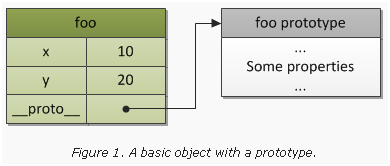## A prototype chain

Prototype对象也是对象类型的，也会有自己的prototypes。如果这个prototype仍然存在一个非空的prototype，那么这样一直搜寻下去，就形成了一个原型链[prototype chain]。

A prototype chain is a finite chain of objects which is used to implemented inheritance and shared properties.

ECMAScript没有类的概念。但是，重用[reuse]这个理念没什么不同（某些方面，甚至比class-更加灵活），可以由prototype chain原型链来实现。这种继承被称为delegation based inheritance-基于继承的委托，或者更通俗一些，叫做原型继承。

```var a = {
x: 10,
calculate: function (z) {
return this.x + this.y + z
}
};

var b = {
y: 20,
__proto__: a
};

var c = {
y: 30,
__proto__: a
};

// call the inherited method
b.calculate(30); // 60
c.calculate(40); // 80```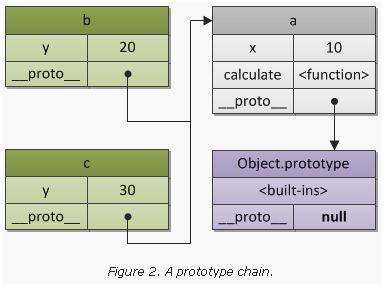## Constructor

```// a constructor function
function Foo(y) {
// which may create objects
// by specified pattern: they have after
// creation own "y" property
this.y = y;
}

// also "Foo.prototype" stores reference
// to the prototype of newly created objects,
// so we may use it to define shared/inherited
// properties or methods, so the same as in
// previous example we have:

// inherited property "x"
Foo.prototype.x = 10;

// and inherited method "calculate"
Foo.prototype.calculate = function (z) {
return this.x + this.y + z;
};

// now create our "b" and "c"
// objects using "pattern" Foo
var b = new Foo(20);
var c = new Foo(30);

// call the inherited method
b.calculate(30); // 60
c.calculate(40); // 80

// let's show that we reference
// properties we expect

console.log(

b.__proto__ === Foo.prototype, // true
c.__proto__ === Foo.prototype, // true

// also "Foo.prototype" automatically creates
// a special property "constructor", which is a
// reference to the constructor function itself;
// instances "b" and "c" may found it via
// delegation and use to check their constructor

b.constructor === Foo, // true
c.constructor === Foo, // true
Foo.prototype.constructor === Foo // true

b.calculate === b.__proto__.calculate, // true
b.__proto__.calculate === Foo.prototype.calculate // true

);```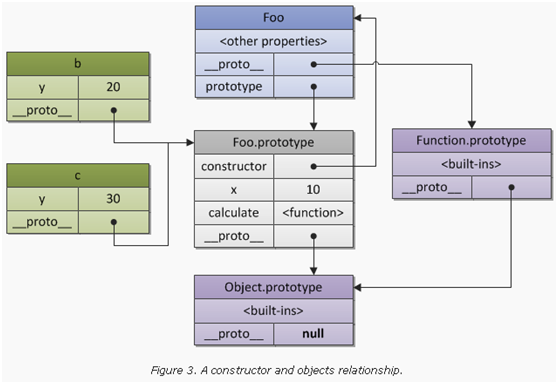## Execution context stack

```function foo(bar) {}

// call the same function,
// generate three different
// contexts in each call, with
// different context state (e.g. value
// of the "bar" argument)

foo(10);
foo(20);
foo(30);```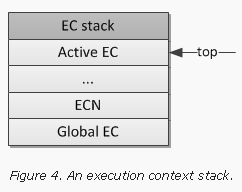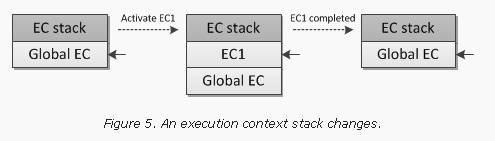## Execution context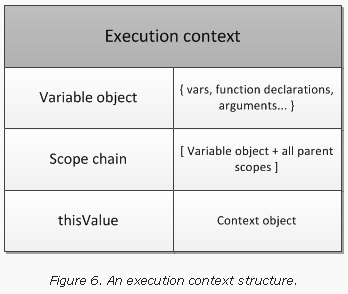## Variable object

A variable object is a scope of data related with the execution context. It’s a special object associated with the context and which stores variables and function declarations are being defined within the context.

Variable Object是一个抽象的概念，某个方面来讲，它表示使用不同的object.以下简称VO. 例如，在global上下文中，variable object也是全局对象[global object]。（这就是我们可以通过全局对象的属性来指向全局变量）。

```var foo = 10;

function bar() {} // function declaration, FD
(function baz() {}); // function expression, FE

console.log(
this.foo == foo, // true
window.bar == bar // true
);

console.log(baz); // ReferenceError, "baz" is not defined```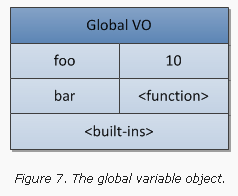## Activation object

```function foo(x, y) {
var z = 30;
function bar() {} // FD
(function baz() {}); // FE
}

foo(10, 20);```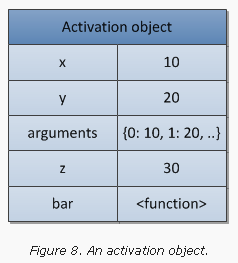## Scope chain

```var x = 10;

(function foo() {
var y = 20;
(function bar() {
var z = 30;
// "x" and "y" are "free variables"
// and are found in the next (after
// bar's activation object) object
// of the bar's scope chain
console.log(x + y + z);
})();
})();```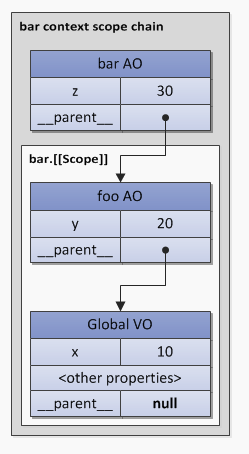1. 首先在原本的作用域链
2. 每一个链接点的作用域的链（如果这个链接点是有prototype的话）

```Object.prototype.x = 10;

var w = 20;
var y = 30;

// in SpiderMonkey global object
// i.e. variable object of the global
// context inherits from "Object.prototype",
// so we may refer "not defined global
// variable x", which is found in
// the prototype chain

console.log(x); // 10

(function foo() {

// "foo" local variables
var w = 40;
var x = 100;

// "x" is found in the
// "Object.prototype", because
// {z: 50} inherits from it

with ({z: 50}) {
console.log(w, x, y , z); // 40, 10, 30, 50
}

// after "with" object is removed
// from the scope chain, "x" is
// again found in the AO of "foo" context;
// variable "w" is also local
console.log(x, w); // 100, 40

// and that's how we may refer
// shadowed global "w" variable in
// the browser host environment
console.log(window.w); // 20

})();```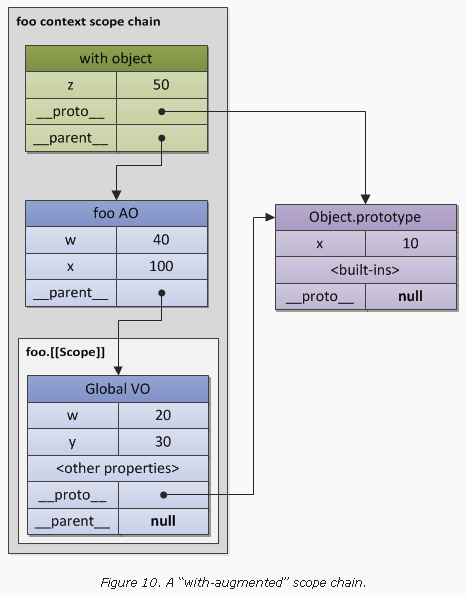## Closure

`Scope chain = Activation object + [[Scope]]`

```function foo() {
var x = 10;
return function bar() {
console.log(x);
};
}

// "foo" returns also a function
// and this returned function uses
// free variable "x"

var returnedFunction = foo();

// global variable "x"
var x = 20;

// execution of the returned function
returnedFunction(); // 10, but not 20```

“funarg problem”的另一个类型就是自上而下[”downward funarg problem”].在这种情况下，父级的上下会存在，但是在判断一个变量值的时候会有多义性。也就是，这个变量究竟应该使用哪个作用域。是在函数创建时的作用域呢，还是在执行时的作用域呢？为了避免这种多义性，可以采用闭包，也就是使用静态作用域。

```// global "x"
var x = 10;

// global function
function foo() {
console.log(x);
}

(function (funArg) {

// local "x"
var x = 20;

// there is no ambiguity,
// because we use global "x",
// which was statically saved in
// [[Scope]] of the "foo" function,
// but not the "x" of the caller's scope,
// which activates the "funArg"

funArg(); // 10, but not 20

})(foo); // pass "down" foo as a "funarg"```

A closure is a combination of a code block (in ECMAScript this is a function) and statically/lexically saved all parent scopes. Thus, via these saved scopes a function may easily refer free variables.

```function baz() {
var x = 1;
return {
foo: function foo() { return ++x; },
bar: function bar() { return --x; }
};
}

var closures = baz();

console.log(
closures.foo(), // 2
closures.bar()  // 1
);```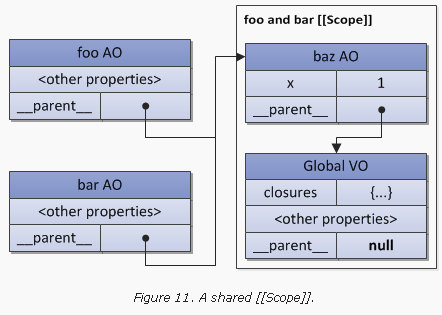```var data = [];

for (var k = 0; k < 3; k++) {
data[k] = function () {
};
}

data(); // 3, but not 0
data(); // 3, but not 1
data(); // 3, but not 2```

```var data = [];

for (var k = 0; k < 3; k++) {
data[k] = (function (x) {
return function () {
};
})(k); // pass "k" value
}

// now it is correct
data(); // 0
data(); // 1
data(); // 2```

## This value

A `this` value is a special object which is related with the execution context. Therefore, it may be named as a context object (i.e. an object in which context the execution context is activated).

this适合执行的上下文环境息息相关的一个特殊对象。因此，它也可以称为上下文对象[context object]

a `this` value is a property of the execution context, but not a property of the variable object.

this是执行上下文环境的一个属性，而不是某个变量对象的属性

```var x = 10;

console.log(
x, // 10
this.x, // 10
window.x // 10
);```

```// the code of the "foo" function
// never changes, but the "this" value
// differs in every activation

function foo() {
}

// caller activates "foo" (callee) and
// provides "this" for the callee

foo(); // global object
foo.prototype.constructor(); // foo.prototype

var bar = {
baz: foo
};

bar.baz(); // bar

(bar.baz)(); // also bar
(bar.baz = bar.baz)(); // but here is global object
(bar.baz, bar.baz)(); // also global object
(false || bar.baz)(); // also global object

var otherFoo = bar.baz;
otherFoo(); // again global object```